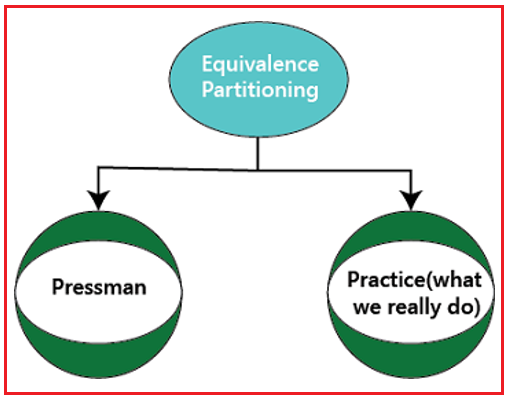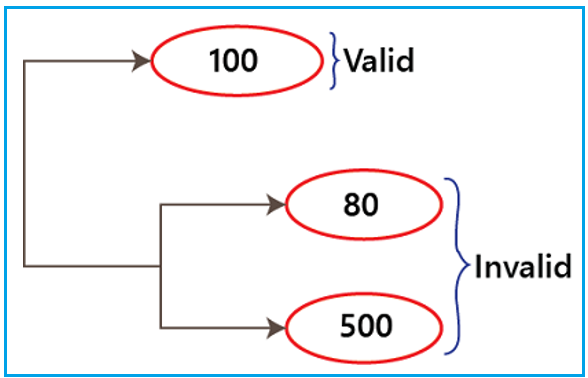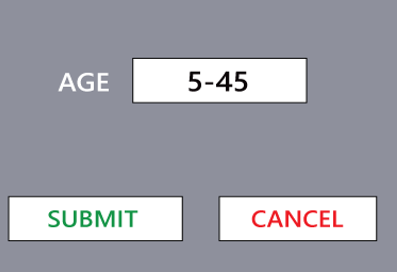# Equivalence Partitioning Techniques in Software Testing

## Equivalence Partitioning Techniques in Software Testing

In this article, I am going to discuss Equivalence Partitioning Techniques in Software Testing. Please read our previous article where we discussed Error Guessing Techniques in Software Testing. At the end of this article, you will understand the following important pointers which are related to Equivalence Partitioning Techniques in Software Testing.

1. What are Equivalence Partitioning Techniques in Software Testing?
2. What is the Identification of Equivalence Classes?
3. How Equivalence Partitioning Technique is Performed in Software Testing?
4. What are the Advantages of Equivalence Partitioning in Testing?
5. What are the Disadvantages of Equivalence Partitioning in Testing?
##### What are Equivalence Partitioning Techniques in Software Testing?

Equivalence class partitioning (ECP) is another name for the Equivalence Partitioning Method. It is a method of software testing known as “BLACK-BOX TESTING” that separates the input domain into classes of data from which test cases can be derived. An ideal test case detects a sort of error that might necessitate the execution of numerous arbitrary test cases before the general error is noticed. Equivalence classes are assessed for predetermined input circumstances in equivalence partitioning. The kind of input condition is confirmed each time an input is given, and for these input conditions, the Equivalence class reflects or specifies a collection of valid or invalid states.

It is a method of software testing that divides the application being tested’s input test data into at least one partition of equivalent data, from which test cases can be produced. Because there are fewer test cases, this method has the benefit of taking less time to complete software testing.

##### What is the Identification of Equivalence Classes?

Let’s talk about some guidelines or best practices for identifying equivalence classes:

• For both positive and negative test scenarios, provide all test data types. All test scenarios must be covered when creating test data classes, however, redundancy of any type must be avoided at all costs.
• It is preferable to split an equivalence class if it is possible that the test data in that class could be handled differently.

For instance, in the example given above, the application is incompatible with numbers less than 10. As a result, we built two classes for numbers 0 through 9, rather than just one class for numbers less than 10. This is due to the potential for the application to treat negative integers in a different manner.

##### How Equivalence Partitioning Technique is Performed in Software Testing?

Equivalence partitioning can be done in one of two approaches, which are as follows:Let’s examine how the pressman and general practice techniques will be used:

Draw the test case for one valid and two invalid inputs if the requirement is a range of values. Here, the Range of values suggests that we always choose equivalence partitioning to obtain the minimum test coverage whenever we want to identify the range values. Then, in order to accomplish the greatest amount of test coverage, we turn to error guessing.

As stated by the pressman: For instance, the amount of test field takes values in the range (100–400):##### According to the General Practice method:

Divide the Range into the internals whenever the need is Range + criteria, then check for each of these values in turn.

For instance: The pressman technique is sufficient in the image below to test for one valid and two invalid entries in the age text field. However, if the age text field has numerous policies for different age groups and the requirement that insurance be held for at least ten years, we must use the practice technique.##### What are the Advantages of Equivalence Partitioning in Testing?
1. Equivalence class testing allows for a significant reduction in test cases while yet retaining the same level of test coverage.
2. This testing method facilitates the quick delivery of high-quality products.
3. It is ideal for software projects with limited resources and time.
##### What are the Disadvantages of Equivalence Partitioning in Testing?
1. Identification of equivalence classes is essential for the overall success of equivalence class testing. The ability of the testers who design these classes and the test cases based on them is what determines which classes can be identified.
2. Finding every set of equivalence classes in the case of complicated applications is quite challenging and necessitates a high level of skill on the tester’s part.
3. Equivalence classes that are incorrectly identified may have lower test coverage and a higher risk of defect leakage.

In the next article, I am going to discuss Boundary Value Analysis Techniques in Software Testing. Here, in this article, I try to explain Equivalence Partitioning Techniques in Software Testing. I hope you enjoy this Equivalence Partitioning Techniques in Software Testing article.